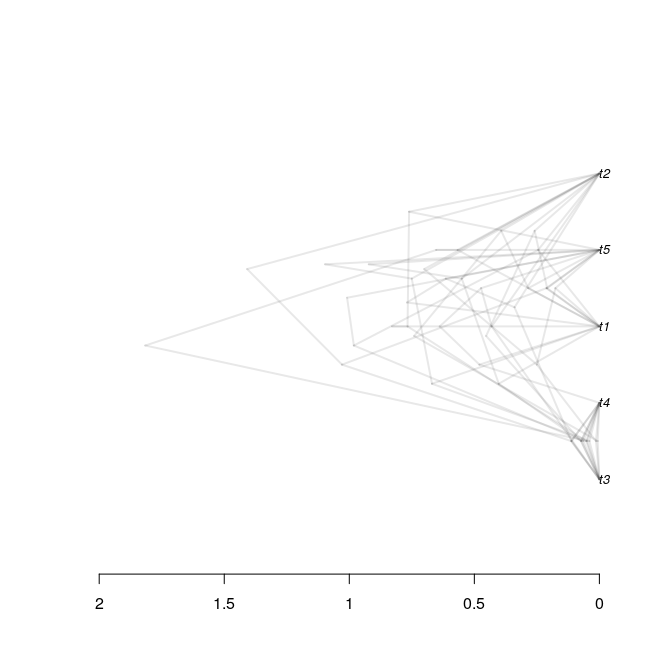# tracerer demo

#### 2019-12-02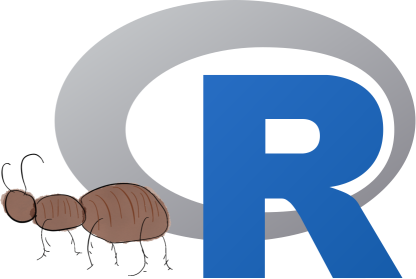tracerer: ‘Tracer for R’ is an R package that does the same as Tracer does, from within R.

To use tracerer, it needs to be loaded:

library(tracerer)

When loading beast2_example_output.log in Tracer, the following is displayed: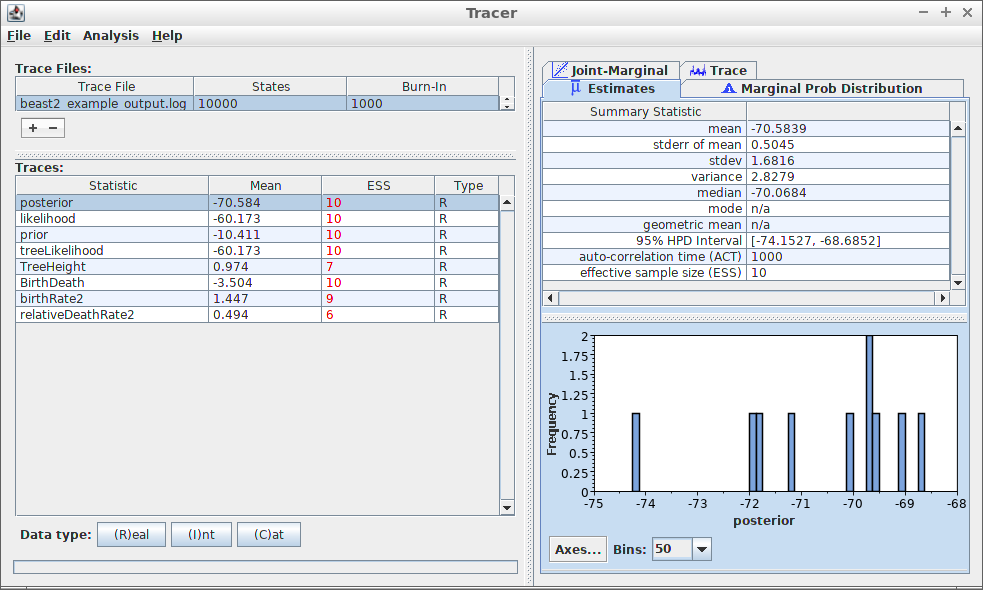Tracer output

Most prominently, at the left, the effective sample sizes (ESSes) are shown.

The show the ESSes using tracerer:

estimates <- parse_beast_log(
get_tracerer_path("beast2_example_output.log")
)
estimates <- remove_burn_ins(estimates, burn_in_fraction = 0.1)
esses <- calc_esses(estimates, sample_interval = 1000)
table <- t(esses)
colnames(table) <- c("ESS")
knitr::kable(table)
ESS
posterior 10
likelihood 10
prior 10
treeLikelihood 10
TreeHeight 7
BirthDeath 10
birthRate2 9
relativeDeathRate2 6

At the top-right, some measures of the variable posterior is shown. To reproduce these measures in tracerer:

sum_stats <- calc_summary_stats(
estimates\$posterior,
sample_interval = 1000
)
table <- t(sum_stats)
colnames(table) <- c("sum_stat")
knitr::kable(table)
sum_stat
mean -70.58394
stderr_mean 0.5044887
stdev 1.681629
variance 2.827876
mode n/a
geom_mean n/a
hpd_interval_low -74.15268
hpd_interval_high -68.68523
act 1000
ess 10

Unlike Tracer, in tracerer all summary statistics can be obtained at once:

sum_stats <- calc_summary_stats(
estimates,
sample_interval = 1000
)
knitr::kable(sum_stats)
mean stderr_mean stdev variance mode geom_mean hpd_interval_low hpd_interval_high act ess
posterior -70.5839432 0.5044887 1.6816291 2.8278764 n/a n/a -74.1526820 -68.6852294 1000.000 10.000000
likelihood -60.1725009 0.3964208 1.3214025 1.7461047 n/a n/a -62.4090389 -58.7371284 1000.000 10.000000
prior -10.4114423 0.5424505 1.8081684 3.2694729 n/a n/a -14.1703653 -7.2820933 1000.000 10.000000
treeLikelihood -60.1725009 0.3964208 1.3214025 1.7461047 n/a n/a -62.4090389 -58.7371284 1000.000 10.000000
TreeHeight 0.9744748 0.1439937 0.3916244 0.1533697 n/a 0.91041547166058 0.4529637 1.8159958 1502.121 6.657254
BirthDeath -3.5036870 0.5424505 1.8081684 3.2694729 n/a n/a -7.2626100 -0.3743380 1000.000 10.000000
birthRate2 1.4470488 0.2134411 0.6713951 0.4507714 n/a 1.28823302868404 0.3909076 2.8041208 1122.942 8.905181
relativeDeathRate2 0.4937568 0.0650235 0.1709096 0.0292101 n/a 0.466468860930895 0.2496224 0.7107459 1608.296 6.217762

At the bottom-right, a histogram of the posterior estimates is shown. To reproduce these measures in tracerer:

ggplot2::ggplot(
data = remove_burn_ins(estimates, burn_in_fraction = 0.1),
ggplot2::aes(posterior)
) + ggplot2::geom_histogram(binwidth = 0.21) + ggplot2::scale_x_continuous(breaks = seq(-75,-68))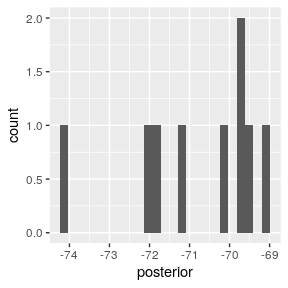Tracer can also show the trace of each estimated variable: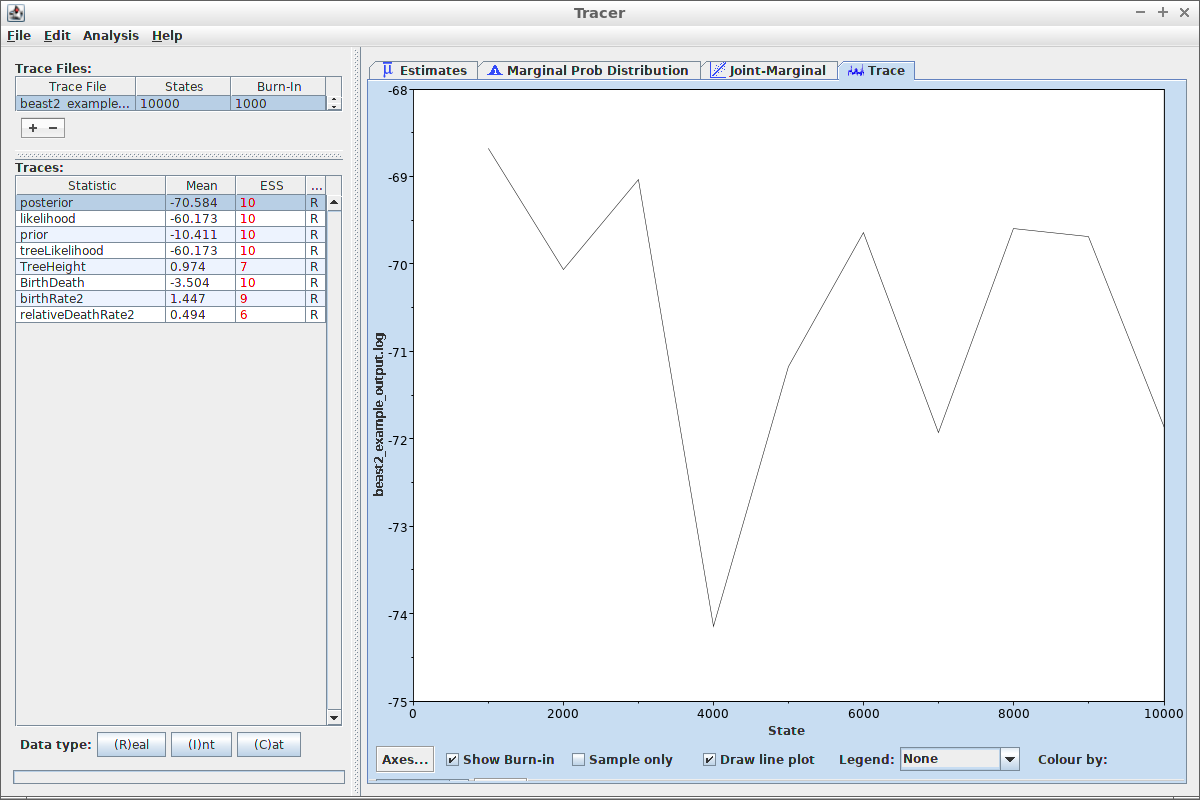Tracer shows the trace of the posterior likelihood

Same can be done with tracerer:

ggplot2::ggplot(
data = remove_burn_ins(estimates, burn_in_fraction = 0.1),
ggplot2::aes(x = Sample)
) + ggplot2::geom_line(ggplot2::aes(y = posterior))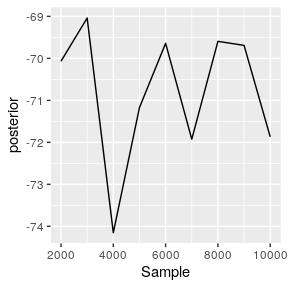tracerer can also use part of DensiTree’s functionality. Here is beast2_example_output.trees displayed by DensiTree: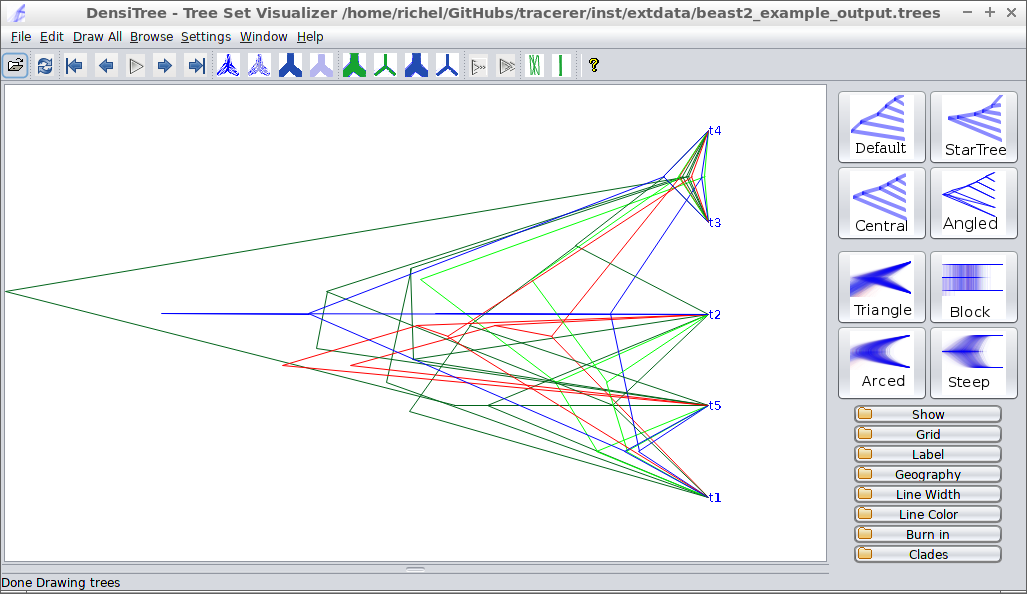DensiTree output

The same is achieved in tracerer with:

trees <- parse_beast_trees(
get_tracerer_path("beast2_example_output.trees")
)
phangorn::densiTree(trees, width = 2)